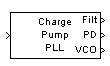# Charge Pump PLL

(To be removed) Implement charge pump phase-locked loop using digital phase detector

Charge Pump PLL will be removed in a future release. To design voltage-controlled oscillators (VCOs) and phase-locked loops (PLLs), use the Phase-Locked Loops (Mixed-Signal Blockset) blocks.

## Library

Components sublibrary of Synchronization

•## Description

The Charge Pump PLL (phase-locked loop) block automatically adjusts the phase of a locally generated signal to match the phase of an input signal. It is suitable for use with digital signals.

This PLL has these three components:

• A sequential logic phase detector, also called a digital phase detector or a phase/frequency detector.

• A filter. You specify the filter transfer function using the Lowpass filter numerator and Lowpass filter denominator parameters. Each is a vector that gives the respective polynomial's coefficients in order of descending powers of s.

To design a filter, use functions such as `butter`, `cheby1`, and `cheby2` in Signal Processing Toolbox™ software. The default filter is a Chebyshev type II filter whose transfer function arises from the command below.

`[num, den] = cheby2(3,40,100,'s')`
• A voltage-controlled oscillator (VCO). You specify characteristics of the VCO using the VCO input sensitivity, VCO quiescent frequency, VCO initial phase, and VCO output amplitude parameters.

This block accepts a sample-based scalar input signal. The input signal represents the received signal. The three output ports produce:

• The output of the filter

• The output of the phase detector

• The output of the VCO

A sequential logic phase detector operates on the zero crossings of the signal waveform. The equilibrium point of the phase difference between the input signal and the VCO signal equals π. The sequential logic detector can compensate for any frequency difference that might exist between a VCO and an incoming signal frequency. Hence, the sequential logic phase detector acts as a frequency detector.

## Parameters

Lowpass filter numerator

The numerator of the lowpass filter transfer function, represented as a vector that lists the coefficients in order of descending powers of s.

Lowpass filter denominator

The denominator of the lowpass filter transfer function, represented as a vector that lists the coefficients in order of descending powers of s.

VCO input sensitivity (Hz/V)

This value scales the input to the VCO and, consequently, the shift from the VCO quiescent frequency value. The units of VCO input sensitivity are Hertz per volt.

VCO quiescent frequency (Hz)

The frequency of the VCO signal when the voltage applied to it is zero. This should match the frequency of the input signal.

The initial phase of the VCO signal.

VCO output amplitude

The amplitude of the VCO signal.